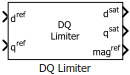# DQ Limiter

Saturate voltages (or current) in the dq reference frame

• Library:
• Motor Control Blockset / Controls / Control Reference

•## Description

The DQ Limiter block generates saturated values of the input voltages (or current) in the dq reference frame, based on the phase voltage (or current) peak limit of the inverter.

The block accepts reference values of d and q axis voltages (or current) and outputs the corresponding saturated values. The block also provides the unsaturated peak value of the reference dq voltages (or current) to enable field weakening control.

### Equations

These equations describe the computation of saturated dq voltage (or current) values by the block.

`$ma{g}^{ref}=\sqrt{{\left({d}^{ref}\right)}^{2}+{\left({q}^{ref}\right)}^{2}}$`

When .

• ${d}^{sat}={d}^{ref}$

• ${q}^{sat}={q}^{ref}$

When .

## Ports

### Input

expand all

Reference voltage (or current) value along the d-axis of the rotating dq reference frame.

Data Types: `single` | `double` | `fixed point`

Reference voltage (or current) value along the q-axis of the rotating dq reference frame.

Data Types: `single` | `double` | `fixed point`

### Output

expand all

Saturated voltage (or current) value along the d-axis of the rotating dq reference frame.

Data Types: `single` | `double` | `fixed point`

Saturated voltage (or current) value along the q-axis of the rotating dq reference frame.

Data Types: `single` | `double` | `fixed point`

Unsaturated peak value of the input voltages (or current).

Data Types: `single` | `double` | `fixed point`

## Parameters

expand all

The maximum magnitude of the stator phase voltage (or current) that the inverter can supply to the motor.

is usually the rated current of the motor. When you work with the Per-Unit system (PU), you should convert the rated current of the motor to Per-Unit value with respect to the base current.

is the maximum phase voltage supplied by the inverter. Generally it is $\frac{{V}_{\text{dc}}}{\sqrt{3}}$ for Space Vector PWM and $\frac{{V}_{\text{dc}}}{2}$ for Sinusoidal PWM, where Vdc is the DC link voltage of the inverter.

Note

You can enter either per unit or SI unit voltage (or current) value in this parameter. For optimum performance, we recommend that you provide a per unit value.

## Version History

Introduced in R2020a